0
9634

# High Level Syllogism Questions for SBI PO 2020 PDF

Download SBI PO High Level Syllogism Questions & Answers PDF for SBI PO Prelims and Mains exam. Top-15 Very Important SBI PO Questions with solutions for Banking Exams.

Instructions

In each question below are given three statements followed by four conclusions I II III and IV You have to take the given statements to be true even if they seem to be at variance with commonly known facts Read all the conclusions and then decide which of the given conclusion logically follow from given statements disregarding commonly Known facts

Question 1: Statements
All towns are villages.
No village is forest.
Some forests are rivers.
Conclusions
I.Some forests are villages.
II.Some forests are not villages.
III.Some rivers are not villages.
IV.All villages are towns.

a) All follow

b) Only either I or II follows

c) Only either I or II and III follows

d) None follow

e) None of these

Instructions

In each of the questions below are given three statements followed by four conclusions numbered I, II, III and IV. You have to take the given statements to be true even if they seem to be at variance from commonly known facts. Read all the conclusions and then decide which of the given conclusions logically follows from the given statements disregarding commonly known facts.

Question 2: Statements: All trains are rivers. Some rivers are houses.
All houses are lakes.
Conclusions:
I. Some lakes are trains
II. Some houses are trains
III. No train is lake
IV. Some houses are rivers

a) None follow

b) Only IV follows

c) Only either I or III follows

d) Only either I or III and IV follow

e) All follow

Question 3: Statements: Some tigers are goats. No goat is rat.
All dogs are rats.
Conclusions:
I. No tiger is rat
II. No dog is goat
III. Some dogs are tigers
IV. Some rats are tigers

a) Only either I or IV and II follow

b) Only either I or IV and III follow

c) Only II follow

d) Only either I or IV follow

e) None follows

Question 4: Statements: Some trees are flowers. Some roads are flowers.
Conclusions:
I. Some vehicles are trees
II. Some vehicles are flowers

a) None follows

b) Only I and II follows

c) Only II follows

d) Only I, III and IV follow

e) All follow

Question 5: Statements: All jungles are buses. All books are buses.
All fruits are books
Conclusions:
I. Some fruits are jungles
II. Some buses are books
III. Some buses are jungles
IV. All fruits are buses

a) All follow

b) Only II, III and IV follow

c) Only I, II and III follow

d) Only I, II and IV follow

e) None of these

SBI PO Free Mock Tests

Question 6: Statements: Some pens are rooms. Some rooms are cats.
Some cats are windows.
Conclusions:
I. Some windows are rooms
II. Some cats are pens
III. Some pens are windows
IV. Some pens are cats

a) All follow

b) Only I follow

c) Only III follow

d) Only II follow

e) None follows

Instructions

In each question below are two statements followed by two conclusions numbered I and II You have to take the two given statements to be true even if they seem to be at variance from known facts and then decide which of the given Conclusions logically commonly follows from the given statements disregarding commonly known facts ?
a:If only Conclusion I follows
b:If only Conclusion II follows
c:If either Conclusion I nor II follows
d:If neither Conclusions I nor II follows
e:If both Conclusion I and II follow

Question 7: Statements Some gears are wheels.All wheels are brakes.
Conclusions
I.No brake is gear.
II.At least some gears are brakes.

a) If only Conclusion I follows

b) If only Conclusion II follows

c) If either Conclusion I nor I follows

d) If neither Conclusions I nor II follows

e) If both Conclusion I and II follow

Question 8: Statements: No month is a year. No year is a second.
Conclusions
I.All months are seconds.
II.No second is month.

a) If only Conclusion I follows

b) If only Conclusion II follows

c) If either Conclusion I nor I follows

d) If neither Conclusions I nor II follows

e) If both Conclusion I and II follow

Question 9: Statements No plane is hill. Some hills are towns.
Conclusions
I.No town is plane.
II.Some towns are plane.

a) If only Conclusion I follows

b) If only Conclusion II follows

c) If either Conclusion I nor I follows

d) If neither Conclusions I nor II follows

e) If both Conclusion I and II follow

Question 10: Statement: All metals are liquids. All liquids are gases
Conclusions:
I.All metals are gases
II.At least some gases are liquids

a) If only Conclusion I follows

b) If only Conclusion II follows

c) If either Conclusion I nor I follows

d) If neither Conclusions I nor II follows

e) If both Conclusion I and II follow

Question 11: Statements: Some cities are towns. Some villages are cities
Conclusions:
I.At least some villages are towns.
II.No village is a town

a) If only Conclusion I follows

b) If only Conclusion II follows

c) If either Conclusion I nor I follows

d) If neither Conclusions I nor II follows

e) If both Conclusion I and II follow

Instructions

In each of the questions below are given three statements followed by four conclusions numbered I, II, III and IV. You have to take the given statements to be true even if they seem to be variance from commonly known facts. Read the conclusion and then decide which of the given conclusions logically follows from the given statements disregarding commonly known facts.

Question 12: Statements: Some dogs are rats
All rats are trees
Some trees are not dogs
Conclusions:
I. Some trees are dogs
II. All dogs are trees
III. All rats are dogs
IV. No trees is dogs

a) None follows

b) Only I follows

c) Only I and II follows

d) Only II and III follows

e) All follow

Question 13: Statements: Some boys are rains
All rains are clouds
Some clouds are cars
Conclusions:
I. Some clouds are boys
II. Some cars are boys
III. Some cars are rains
IV. Some rains are boys

a) None follows

b) Only IV follow

c) Only I follows

d) Both I and IV follow

e) All follow

Question 14: Statements: All bricks are flowers.
Some houses are flowers
All pens are houses
Conclusions:
I. Some house are bricks
II. Some pens are flowers
III. Some flowers are bricks
IV. No pen is flowers

a) Only either II or IV and III follow

b) Only either II or IV and I follow

c) Only either I or III and IV follow

d) None follows

e) All follow

Question 15: Statements: All lions are ducks
No duck is horse
All horses are fruits
Conclusions:
I. No lion is horse
II. Some fruits are horses
III. Some ducks are lions
IV. Some lions are horses

a) All follow

b) Only either I or II and both III and IV follow

c) Only either I or IV and both II and III follow

d) Only either I or IV and II follow

e) None of these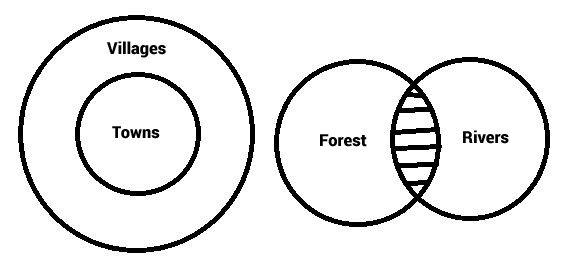Given that no village is a forest. So, the shaded area can never be a village. Hence, some rivers can never be villages. So, conclusion 3 is implied.

Irrespective of the diagram, conclusions I and II are universally complimentary ie. one of the two always has to be implied but both can’t be true at the same time. Hence we use “either” and “or”.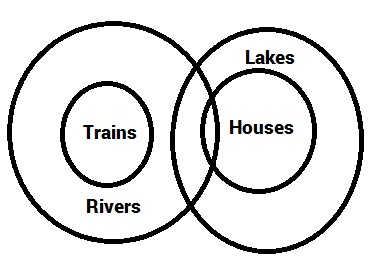We can draw many scenarios using Venn diagrams. Conclusion IV holds in every case. So, it is implied.

Irrespective of the diagram, conclusions I and III are universally complimentary ie. one of the two always has to be implied but both can’t be true at the same time. Hence we use “either” and “or”.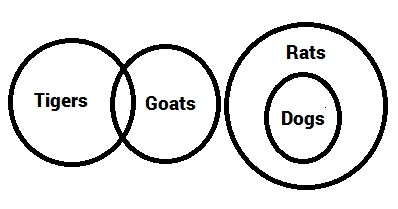No goat is a rat. Hence, as shown in the diagram, none of rats’ subset (dogs) are goats. Hence, conclusion II is implied.

Irrespective of the diagram, conclusions I and IV are universally complimentary ie. one of the two always has to be implied but both can’t be true at the same time. Hence we use “either” and “or”.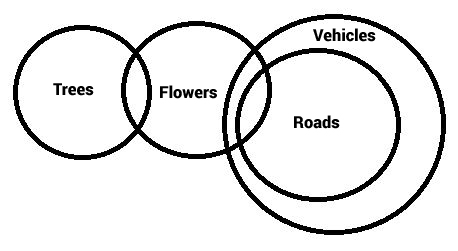We use elimination to find an exception to the generality of the conclusions. Thus we prove they are not implied. The diagram below satisfies all the statements but contradicts the conclusions I, III and IV. Since we found an exception, the conclusions are not true in “every” case. Thus they are not implied.

We can draw many scenarios using Venn diagrams. Conclusion II holds in every case. So, it is implied.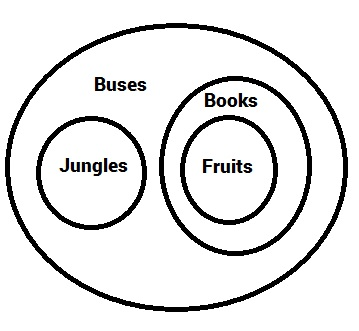We use elimination to find an exception to the generality of the conclusions. Thus we prove they are not implied. The diagram below satisfies all the statements but contradicts conclusion I. Since we found an exception, the conclusion is not true in “every” case. Thus it is not implied.

We can draw many scenarios using Venn diagrams. Conclusions II, III and IV hold in every case. So, they are implied.

We use elimination to find an exception to the generality of the conclusions. Thus we prove they are not implied. The diagram below satisfies all the statements but contradicts all the conclusions. Since we found an exception, the conclusions are not true in “every” case. Thus they are not implied.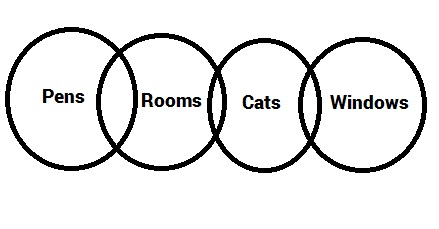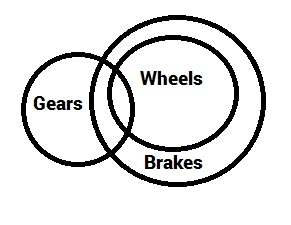We use elimination to find an exception to the generality of the conclusions. Thus we prove they are not implied. The diagram below satisfies all the statements but contradicts the conclusion I. Since we found an exception, the conclusion is not true in “every” case. Thus it is not implied.

We can draw many scenarios using Venn diagrams. Conclusion II holds in every case. So, it is implied.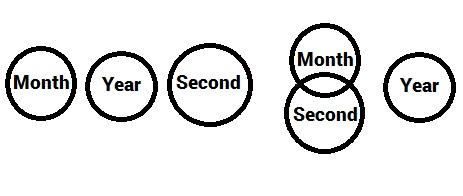Use the left diagram (3 circles on the left) to contradict conclusion I.
Use the right diagram (3 circles on the right) to contradict conclusion II.

We use elimination to find an exception to the generality of the conclusions. Thus we prove they are not implied. The diagrams above satisfy all the statements but contradict both the conclusions. Since we found an exception, the conclusions are not true in “every” case. Thus they are not implied.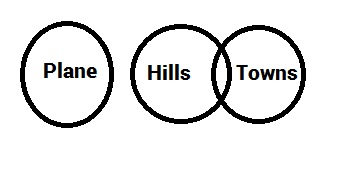Irrespective of the diagram, conclusions I and II are universally complimentary ie. one of the two always has to be implied but both can’t be true at the same time. Hence we use “either” and “or”.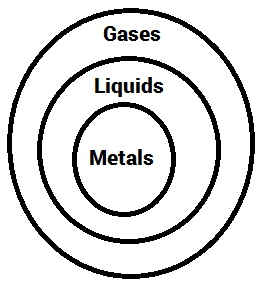We can draw many scenarios using Venn diagrams that satisy the statements and check for the conclusions. Conclusions I and II hold in every case. So, they are implied.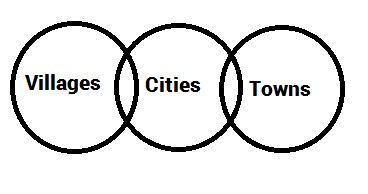Irrespective of the diagram, conclusions I and II are universally complimentary ie. one of the two always has to be implied but both can’t be true at the same time. Hence we use “either” and “or”.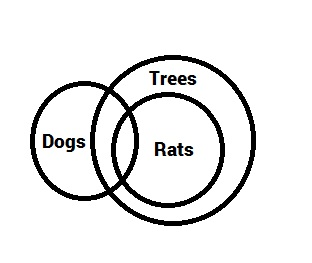We use elimination to find an exception to the generality of the conclusions. Thus we prove they are not implied. The diagram above satisfies all the statements but contradicts all the conclusions except I. Since we found an exception, the conclusions are not true in “every” case. Thus they are not implied.

We can draw many scenarios that satisfy the statements using Venn diagrams and check for the validity of the conclusions. Conclusion I holds in every case. So, it is implied.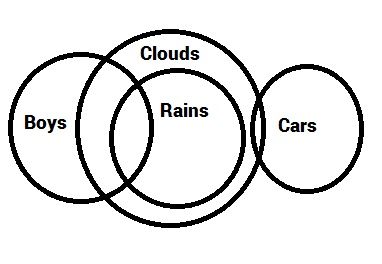We use elimination to find an exception to the generality of the conclusions. Thus we prove they are not implied. The diagram above satisfies all the statements but contradicts the conclusions II and III. Since we found an exception, the conclusions are not true in “every” case. Thus they are not implied.

We can draw many scenarios that satisfy the statements using Venn diagrams and check for the validity of the conclusions. Conclusions I and IV hold in every case. So, they are implied.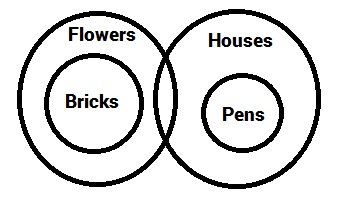We can draw many scenarios that satisfy the statements using Venn diagrams and check for the validity of the conclusions. Conclusion III holds in every case. So, it is implied.
Irrespective of the diagram, conclusions II and IV are universally complimentary ie. one of the two always has to be implied but both can’t be true at the same time. Hence we use “either” and “or”.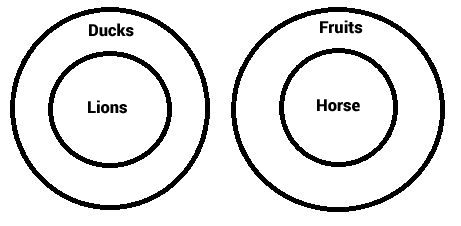We use elimination to find an exception to the generality of the conclusions. Thus we prove they are not implied. The diagram above satisfies all the statements but contradicts the conclusion IV. Since we found an exception, the conclusion is not true in “every” case. Thus it is not implied.